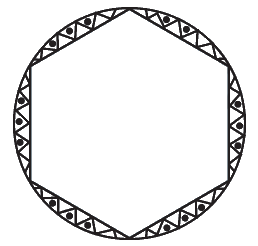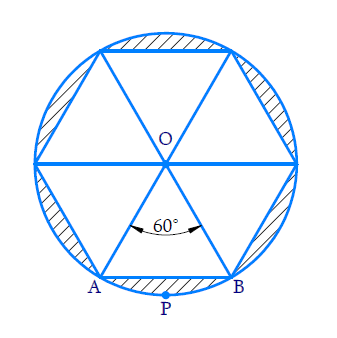# Ex.12.2 Q13 Areas Related to Circles Solution - NCERT Maths Class 10

## Question

A round table cover has six equal designs as shown in figure. If the radius of the cover is $${28 \,\rm{cm},}$$ find the cost of making the designs at the rate of

$$\rm{₹}\, 0.35$$per $$\rm{cm^2.}$$(Use$$\sqrt{3}={ }1.7$$ )Video Solution
Areas Related To Circles
Ex 12.2 | Question 13

## Text Solution

What is known?

A round table cover has $$6$$ equal designs as shown in the figure. The radius of the cover $$= 28\, \rm{ cm}$$and the rate of making design is $$\rm{₹\; 0.35}$$ per $$\rm{cm^2}$$

What is unknown?

The cost of making the design.

Reasoning:

In a circle with radius r and angle at the center with degree measure$$\theta$$

(i) Area of the sector\begin{align} = \frac{{\rm{\theta }}}{{{{360}^{\rm{o}}}}} \times \pi {r^2}\end{align}

(ii) Area of the segment = Area of the sector - Area of the corresponding triangle.(i) Visually it is clear that the designs are segments of the circle

$$\therefore\;$$Area of the design $$=$$ Area of $$6$$segments of the circle.

(ii) Since the table cover has $$6$$ equal design therefore angle of each segment at the center\begin{align} = \frac{{{{360}^o}}}{6} = {60^o}\end{align}

(iii) Consider segment $${APB.}$$Chord $${AB}$$ subtends an angle of $${60^\circ}$$ at the centre.

$$\therefore\;$$ Area of segment$${APB} =$$Area of sector $${AOPB}\, -$$ Area of $$\Delta {AOB}$$

(iv) To find area of $$\Delta AOB$$

In $$\Delta {AOB}$$

$${OA = OB}$$ (radii of the circle)

\begin{align}\angle {OAB} = \angle {OBA} \end{align} (angles oppositeequal sides of a triangle are equal)

\begin{align}\angle {AOB} + \angle {OAB} + \angle {OBA} = {180}^\circ\end{align} (Using angle sum property of a triangle)}

\begin{align}\angle AOB + 2\angle OAB &= {{180}^\circ }\\2\angle OAB &= {180^\circ } - {60^\circ }\\ \!\!&= 120^\circ \\ \angle OAB &= \frac{{120}}{2} \\&= {60^\circ }\\ &= \angle OBA\end{align}

Since all angles of a triangle are of measure $${60^\circ}$$

$$\therefore\;\Delta \,{AOB}$$is an equilateral triangle.

Using area of equilateral triangle \begin{align}=\frac{{\sqrt 3 }}{4}{\left( \rm{side} \right)^2}\end{align}

Area of\begin{align}\Delta AOB = \frac{{\sqrt 3 }}{4}{r^2}\end{align}

Area of sector \begin{align}{{AOBP = }}\frac{{{{6}}{{{0}}^{{\circ}}}}}{{{{36}}{{{0}}^{{\circ}}}}}\times \pi {{{r}}^{{2}}}\end{align}

Area of Segment APB \begin{align}{\rm{ = }}\frac{{{{60}^o}}}{{{{360}^o}}}\pi {r^2} - \frac{{\sqrt 3 }}{4}{r^2}\end{align}

Area of designs \begin{align}{\rm{ = 6}} \times \left( {\frac{{{{60}^o}}}{{{{360}^o}}}\pi {r^2} - \frac{{\sqrt 3 }}{4}{r^2}} \right)\end{align}

Since we know cost of making $$1\,\rm{cm}^2$$of designs we can use unitary method to find cost of designs.

Steps:From the figure we observe the designs are made in the segments of a circle.

Since the table cover has $$6$$ equal designs

$$\therefore\;$$angle subtended by each chord (bounding the segment) at the center \begin{align}= \frac{{{{360}^ \circ }}}{6} = {60^ \circ }\end{align}

Consider $$\Delta AOB$$

$$\Delta {OAB}\,=\,\Delta {OBA }$$($${QOB = OA,}$$angles opposite equal sides in a triangle are equal)

$$\Delta {AOB}\,{+}\,\Delta {OAB}\,{+}\,\,\Delta {OBA}\,{=18}{{{0}}^{{o}}}$$ (angle sum of a triangle)

\begin{align}{2}\Delta {OAB}\,&{=}\,{18}{{{0}}^{{o}}}-{6}{{{0}}^{{o}}} \\ \Delta {OAB}\,\,&{=}\,\frac{{12}{{{0}}^{{o}}}}{{2}} \\ & {=6}{{{0}}^{{o}}} \end{align}

$$\therefore\;\Delta {AOB}$$is an equilateral triangle

Area of $$\Delta AOB$$

\begin{align}&= \frac{{\sqrt 3 }}{4}{({\rm{side}})^2}\\ &= \frac{{\sqrt 3 }}{4} \times {(28)^2}\\ &= \sqrt 3 \times 7 \times 28\\& = 196\sqrt 3 \\ &= 196 \times 1.7\\& = 333.2\,\rm{cm}^2\end{align}

Area of sector $$OAPB$$

\begin{align}&=\,\frac{{{{6}}{{{0}}^{{o}}}}}{{{{36}}{{{0}}^{{o}}}}}{\times}\,{\pi }{{{r}}^{{2}}}\\\,&= \,\frac{{{1}}}{{{6}}}\,{\times }\,\frac{{{{22}}}}{{{7}}}\,{\times}\,{{28}}\,{\times }\,{{28}}\\\,\,& = \,\frac{{{{11} \times 4 \times 28}}}{{{3}}}\\\, &= \,\frac{{{{1232}}}}{{{3}}}\,\rm{{cm}^{{2}}}\end{align}

Area of segment $${APB =}$$ Area of sector $${OAPB}\, -$$Area of $$\Delta {AOB}$$

\begin{align} &= \left( {\frac{{1232}}{3} - 333.2} \right)\,\rm{cm^2}\end{align}

Area of designs $$= 6$$ Area of segment

\begin{align} &= 6 \times\!\!{ }\left( \frac{{1232}}{{3}}{-333}{.2} \right) \\ &= 2464 - 1999.2\,\rm{c}{{{m}}^{{2}}} \\ \\ &= 464{.8}\,\rm{c}{{{m}}^{{2}}} \end{align}

Cost of making $$1\, \rm{cm}^2$$of designs $$\rm{= ₹\; 0.35}$$

$$\therefore\;$$Cost of making $$464.8\,\rm {cm}^2$$ of designs

$$= \rm{₹}\,0.35 \times 464.8\\ =\rm{₹}\, 162.68$$

Learn from the best math teachers and top your exams

• Live one on one classroom and doubt clearing
• Practice worksheets in and after class for conceptual clarity
• Personalized curriculum to keep up with school